2017-03-31 08:30:54 xuena_xiaoming 阅读数 2263
• ###### 机器学习入门30天实战

购买课程后，可扫码进入学习群，获取唐宇迪老师答疑 系列课程包含Python机器学习库，机器学习经典算法原理推导，基于真实数据集案例实战3大模块。从入门开始进行机器学习原理推导，以通俗易懂为基础形象解读晦涩难懂的机器学习算法工作原理，案例实战中使用Python工具库从数据预处理开始一步步完成整个建模工作！具体内容涉及Python必备机器学习库、线性回归算法原理推导、Python实现逻辑回归与梯度下降、案例实战，信用卡欺诈检测、决策树与集成算法、支持向量机原理推导、SVM实例与贝叶斯算法、机器学习常规套路与Xgboost算法、神经网络。

7599 人正在学习 去看看 唐宇迪

1、常用的统计量

``````import numpy as np
from scipy import stats
import math
import matplotlib as mpl
import matplotlib.pyplot as plt
from mpl_toolkits.mplot3d import Axes3D
from matplotlib import cm

def calc_statistics(x):
n = x.shape  # 样本个数

# 手动计算
m = 0
m2 = 0
m3 = 0
m4 = 0
for t in x:
m += t
m2 += t*t
m3 += t**3
m4 += t**4
m /= n
m2 /= n
m3 /= n
m4 /= n

mu = m
sigma = np.sqrt(m2 - mu*mu)
skew = (m3 - 3*mu*m2 + 2*mu**3) / sigma**3
kurtosis = (m4 - 4*mu*m3 + 6*mu*mu*m2 - 4*mu**3*mu + mu**4) / sigma**4 - 3
print '手动计算均值、标准差、偏度、峰度：', mu, sigma, skew, kurtosis

# 使用系统函数验证
mu = np.mean(x, axis=0)
sigma = np.std(x, axis=0)
skew = stats.skew(x)
kurtosis = stats.kurtosis(x)
return mu, sigma, skew, kurtosis

if __name__ == '__main__':
d = np.random.randn(1000)
print d
mu, sigma, skew, kurtosis = calc_statistics(d)
print '函数库计算均值、标准差、偏度、峰度：', mu, sigma, skew, kurtosis
# 一维直方图
mpl.rcParams[u'font.sans-serif'] = 'SimHei'
mpl.rcParams[u'axes.unicode_minus'] = False
y1, x1, dummy = plt.hist(d, bins=50, normed=True, color='g', alpha=0.75)
t = np.arange(x1.min(), x1.max(), 0.05)
y = np.exp(-t**2 / 2) / math.sqrt(2*math.pi)
plt.plot(t, y, 'r-', lw=2)
plt.title(u'高斯分布，样本个数：%d' % d.shape)
plt.grid(True)
plt.show()

d = np.random.randn(1000, 2)
mu, sigma, skew, kurtosis = calc_statistics(d)
print '函数库计算均值、标准差、偏度、峰度：', mu, sigma, skew, kurtosis

# 二维图像
N = 30
density, edges = np.histogramdd(d, bins=[N, N])
print '样本总数：', np.sum(density)
density /= density.max()
x = y = np.arange(N)
t = np.meshgrid(x, y)
fig = plt.figure(facecolor='w')
ax.scatter(t, t, density, c='r', s=15*density, marker='o', depthshade=True)
ax.plot_surface(t, t, density, cmap=cm.Accent, rstride=2, cstride=2, alpha=0.9, lw=0.75)
ax.set_xlabel(u'X')
ax.set_ylabel(u'Y')
ax.set_zlabel(u'Z')
plt.title(u'二元高斯分布，样本个数：%d' % d.shape, fontsize=20)
plt.tight_layout(0.1)
plt.show()``````

[ 1.02372188e+00 -7.94235491e-01 -9.98698986e-01 …, -9.98193952e-01 5.28687536e-01 -1.89125334e+00]

python提供了直接计算的封装包，和我们手动计算的结果一致，如果有需求直接调用封装包即可。2、fft
fft的主要功能是进行时域频域信号转换，比如三角波信号从频域恢复到时域，如下图示：``````import numpy as np
import matplotlib as mpl
import matplotlib.pyplot as plt

def triangle_wave(size, T):
t = np.linspace(-1, 1, size, endpoint=False)
# where
# y = np.where(t < 0, -t, 0)
# y = np.where(t >= 0, t, y)
y = np.abs(t)
y = np.tile(y, T) - 0.5
x = np.linspace(0, 2*np.pi*T, size*T, endpoint=False)
return x, y

def sawtooth_wave(size, T):
t = np.linspace(-1, 1, size)
y = np.tile(t, T)
x = np.linspace(0, 2*np.pi*T, size*T, endpoint=False)
return x, y

def triangle_wave2(size, T):
x, y = sawtooth_wave(size, T)
return x, np.abs(y)

def non_zero(f):
f1 = np.real(f)
f2 = np.imag(f)
eps = 1e-4
return f1[(f1 > eps) | (f1 < -eps)], f2[(f2 > eps) | (f2 < -eps)]

if __name__ == "__main__":
mpl.rcParams['font.sans-serif'] = [u'simHei']
mpl.rcParams['axes.unicode_minus'] = False
np.set_printoptions(suppress=True)

x = np.linspace(0, 2*np.pi, 16, endpoint=False)
print '时域采样值：', x
y = np.sin(2*x) + np.sin(3*x + np.pi/4) + np.sin(5*x)
# y = np.sin(x)

N = len(x)
print '采样点个数：', N
print '\n原始信号：', y
f = np.fft.fft(y)
print '\n频域信号：', f/N
a = np.abs(f/N)
print '\n频率强度：', a

iy = np.fft.ifft(f)
print '\n逆傅里叶变换恢复信号：', iy
print '\n虚部：', np.imag(iy)
print '\n实部：', np.real(iy)
print '\n恢复信号与原始信号是否相同：', np.allclose(np.real(iy), y)

plt.subplot(211)
plt.plot(x, y, 'go-', lw=2)
plt.title(u'时域信号', fontsize=15)
plt.grid(True)
plt.subplot(212)
w = np.arange(N) * 2*np.pi / N
print u'频率采样值：', w
plt.stem(w, a, linefmt='r-', markerfmt='ro')
plt.title(u'频域信号', fontsize=15)
plt.grid(True)
plt.show()

# 三角/锯齿波
x, y = triangle_wave(20, 5)
# x, y = sawtooth_wave(20, 5)
N = len(y)
f = np.fft.fft(y)
# print '原始频域信号：', np.real(f), np.imag(f)
print '原始频域信号：', non_zero(f)
a = np.abs(f / N)

# np.real_if_close
f_real = np.real(f)
eps = 0.3 * f_real.max()
print eps
f_real[(f_real < eps) & (f_real > -eps)] = 0
f_imag = np.imag(f)
eps = 0.3 * f_imag.max()
print eps
f_imag[(f_imag < eps) & (f_imag > -eps)] = 0
f1 = f_real + f_imag * 1j
y1 = np.fft.ifft(f1)
y1 = np.real(y1)
# print '恢复频域信号：', np.real(f1), np.imag(f1)
print '恢复频域信号：', non_zero(f1)

plt.figure(figsize=(8, 8), facecolor='w')
plt.subplot(311)
plt.plot(x, y, 'g-', lw=2)
plt.title(u'三角波', fontsize=15)
plt.grid(True)
plt.subplot(312)
w = np.arange(N) * 2*np.pi / N
plt.stem(w, a, linefmt='r-', markerfmt='ro')
plt.title(u'频域信号', fontsize=15)
plt.grid(True)
plt.subplot(313)
plt.plot(x, y1, 'b-', lw=2, markersize=4)
plt.title(u'三角波恢复信号', fontsize=15)
plt.grid(True)
plt.tight_layout(1.5, rect=[0, 0.04, 1, 0.96])
plt.suptitle(u'快速傅里叶变换FFT与频域滤波', fontsize=17)
plt.show()``````

3、SVD

``````import numpy as np
import os
from PIL import Image
import matplotlib.pyplot as plt
import matplotlib as mpl
from pprint import pprint

def restore1(sigma, u, v, K):  # 奇异值、左特征向量、右特征向量
m = len(u)
n = len(v)
a = np.zeros((m, n))
for k in range(K):
uk = u[:, k].reshape(m, 1)
vk = v[k].reshape(1, n)
a += sigma[k] * np.dot(uk, vk)
a[a < 0] = 0
a[a > 255] = 255
# a = a.clip(0, 255)
return np.rint(a).astype('uint8')

def restore2(sigma, u, v, K):  # 奇异值、左特征向量、右特征向量
m = len(u)
n = len(v)
a = np.zeros((m, n))
for k in range(K+1):
for i in range(m):
a[i] += sigma[k] * u[i][k] * v[k]
a[a < 0] = 0
a[a > 255] = 255
return np.rint(a).astype('uint8')

if __name__ == "__main__":
A = Image.open("me.png", 'r')
print A
output_path = r'.\Pic'
if not os.path.exists(output_path):
os.mkdir(output_path)
a = np.array(A)
print a.shape
K = 50
u_r, sigma_r, v_r = np.linalg.svd(a[:, :, 0])
u_g, sigma_g, v_g = np.linalg.svd(a[:, :, 1])
u_b, sigma_b, v_b = np.linalg.svd(a[:, :, 2])
plt.figure(figsize=(10,10), facecolor='w')
mpl.rcParams['font.sans-serif'] = [u'simHei']
mpl.rcParams['axes.unicode_minus'] = False
for k in range(1, K+1):
print k
R = restore1(sigma_r, u_r, v_r, k)
G = restore1(sigma_g, u_g, v_g, k)
B = restore1(sigma_b, u_b, v_b, k)
I = np.stack((R, G, B), axis=2)
Image.fromarray(I).save('%s\\svd_%d.png' % (output_path, k))
if k <= 12:
plt.subplot(3, 4, k)
plt.imshow(I)
plt.axis('off')
plt.title(u'奇异值个数：%d' % k)
plt.suptitle(u'SVD与图像分解', fontsize=20)
plt.tight_layout(0.3, rect=(0, 0, 1, 0.92))
plt.show()``````4、股票k线图

``````import numpy as np
import matplotlib as mpl
import matplotlib.pyplot as plt
from matplotlib.finance import candlestick_ohlc

if __name__ == "__main__":
mpl.rcParams['font.sans-serif'] = [u'SimHei']
mpl.rcParams['axes.unicode_minus'] = False

np.set_printoptions(suppress=True, linewidth=100, edgeitems=5)
data = np.loadtxt('SH600000.txt', dtype=np.float, delimiter='\t', skiprows=2, usecols=(1, 2, 3, 4))
data = data[:50]
N = len(data)

t = np.arange(1, N+1).reshape((-1, 1))
data = np.hstack((t, data))

fig, ax = plt.subplots(facecolor='w')
candlestick_ohlc(ax, data, width=0.6, colorup='r', colordown='g', alpha=0.9)
plt.xlim((0, N+1))
plt.grid(b=True)
plt.title(u'股票K线图', fontsize=18)
plt.tight_layout(2)
plt.show()``````5、分形

``````#!/usr/bin/python
# -*- coding:utf-8 -*-
__author__ = 'xuena'

import numpy as np
import pylab as pl
import time
from matplotlib import cm

def iter_point(c):
z = c
for i in xrange(1, 100): # 最多迭代100次
if abs(z)>2: break # 半径大于2则认为逃逸
z = z*z+c
return i # 返回迭代次数

def draw_mandelbrot(cx, cy, d):
"""
绘制点(cx, cy)附近正负d的范围的Mandelbrot
"""
x0, x1, y0, y1 = cx-d, cx+d, cy-d, cy+d
y, x = np.ogrid[y0:y1:200j, x0:x1:200j]
c = x + y*1j
start = time.clock()
mandelbrot = np.frompyfunc(iter_point,1,1)(c).astype(np.float)
print "time=",time.clock() - start
pl.imshow(mandelbrot, cmap=cm.jet, extent=[x0,x1,y0,y1])
pl.gca().set_axis_off()

x,y = 0.27322626, 0.595153338

pl.subplot(231)
draw_mandelbrot(-0.5,0,1.5)
for i in range(2,7):
pl.subplot(230+i)
draw_mandelbrot(x, y, 0.2**(i-1))
pl.subplots_adjust(0.02, 0, 0.98, 1, 0.02, 0)
pl.show()``````k线 机器学习 相关内容

2019-12-16 23:34:15 weixin_42156097 阅读数 5
• ###### 机器学习入门30天实战

购买课程后，可扫码进入学习群，获取唐宇迪老师答疑 系列课程包含Python机器学习库，机器学习经典算法原理推导，基于真实数据集案例实战3大模块。从入门开始进行机器学习原理推导，以通俗易懂为基础形象解读晦涩难懂的机器学习算法工作原理，案例实战中使用Python工具库从数据预处理开始一步步完成整个建模工作！具体内容涉及Python必备机器学习库、线性回归算法原理推导、Python实现逻辑回归与梯度下降、案例实战，信用卡欺诈检测、决策树与集成算法、支持向量机原理推导、SVM实例与贝叶斯算法、机器学习常规套路与Xgboost算法、神经网络。

7599 人正在学习 去看看 唐宇迪

# 机器学习传统算法概要

## 如何选择合适的算法

k线 机器学习 相关内容

2018-09-27 14:01:07 qq_33361618 阅读数 2195
• ###### 机器学习入门30天实战

购买课程后，可扫码进入学习群，获取唐宇迪老师答疑 系列课程包含Python机器学习库，机器学习经典算法原理推导，基于真实数据集案例实战3大模块。从入门开始进行机器学习原理推导，以通俗易懂为基础形象解读晦涩难懂的机器学习算法工作原理，案例实战中使用Python工具库从数据预处理开始一步步完成整个建模工作！具体内容涉及Python必备机器学习库、线性回归算法原理推导、Python实现逻辑回归与梯度下降、案例实战，信用卡欺诈检测、决策树与集成算法、支持向量机原理推导、SVM实例与贝叶斯算法、机器学习常规套路与Xgboost算法、神经网络。

7599 人正在学习 去看看 唐宇迪

Python机器学习主要调用模块为sklearn，里面有机器学习使用的各种模型算法以及评价指标。

• 特定复杂度的模型对较小的数据集容易过拟合，此时训练集的得分较高，检验集的得分较低；
• 特定复杂度的模型对较大的数据集容易欠拟合；随着数据的增大，训练集得分会不断降低，检验集评分会不断升高；
• 模型的检验集得分永远不会高于训练集得分，两条曲线不断靠近，却不会交叉。

（一）构造多项式回归模型，生成测试样本数据。

``````#构造多项式模型
from sklearn.preprocessing import PolynomialFeatures #专门产生多项式的工具并且包含相互影响的特征集
from sklearn.linear_model import LinearRegression #线性回归模型
from sklearn.pipeline import make_pipeline #构造管道

def PolynomialRegression(degree=2, **kwargs): #**kwargs 形参，返回值为字典类型
return make_pipeline(PolynomialFeatures(degree),
LinearRegression(**kwargs))

#形成样本数据

import numpy as np

def make_data(N, err=1.0, rseed=1):
#随机抽样数据
rng = np.random.RandomState(rseed)
X = rng.rand(N, 1) ** 2
y = 10 - 1./ (X.ravel() + 0.1)
if err > 0:
y += err * rng.randn(N)
return X, y
X, y = make_data(40)``````

（二）绘制多项式函数图像

``````%matplotlib inline
import matplotlib.pyplot as plt
import seaborn; seaborn.set() #设置图形样式

X_test = np.linspace(-0.1, 1.1, 500)[:, None]

plt.scatter(X.ravel(), y, color='black')
axis = plt.axis()
for degree in [1, 3, 5]:
y_test = PolynomialRegression(degree).fit(X,y).predict(X_test)
plt.plot(X_test.ravel(), y_test, label='degree={0}'.format(degree))
plt.xlim(-0.1, 1.0)
plt.ylim(-2, 12)
plt.legend(loc='best');``````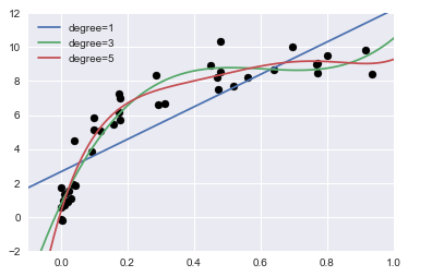（三）绘制学习曲线

``````from sklearn.learning_curve import validation_curve

degree = np.arange(0, 21)
train_score,val_score = validation_curve(PolynomialRegression(), X, y,
'polynomialfeatures__degree',
degree, cv=7)
plt.plot(degree, np.median(train_score, 1),color='blue', label='training score')
plt.plot(degree, np.median(val_score, 1), color='red', label='validation score')
plt.legend(loc='best')
plt.ylim(0, 1)
plt.xlabel('degree')
plt.ylabel('score')``````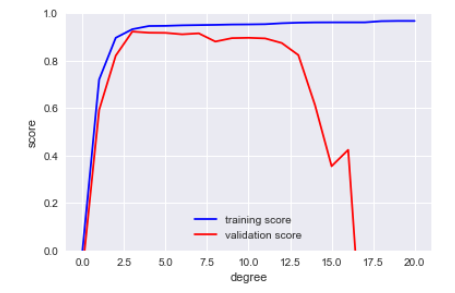结果显示：随着模型复杂度的增加，训练得分（图中的蓝线部分）快速增加，达到饱和之后，增长幅度较低，趋于平缓；

（四）增加样本数量，观察模型拟合效果

``````X2, y2 = make_data(200) #生成200个数据样本
plt.scatter(X2.ravel(), y2)

#重新绘制学习曲线，并将小样本曲线添加上去

degree = np.arange(21)
train_score2, val_score2 = validation_curve(PolynomialRegression(), X2, y2,
'polynomialfeatures__degree',
degree, cv=7)

plt.plot(degree, np.median(train_score2, 1), color='blue',
label='train score')
plt.plot(degree, np.median(val_score2, 1), color='red',
label='validation score')
plt.plot(degree, np.median(train_score, 1),color='blue', alpha=0.3,
linestyle='dashed')
plt.plot(degree, np.median(val_score, 1), color='red', alpha=0.3,
linestyle='dashed')
plt.legend(loc='lower center')
plt.ylim(0, 1)
plt.xlabel('degree')
plt.ylabel('score')``````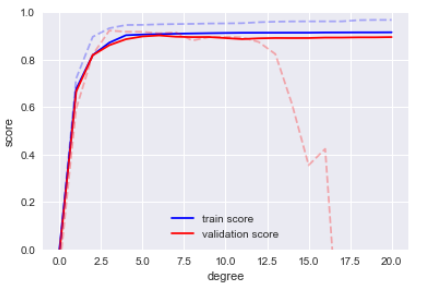其中虚线代表小数据集的学习曲线，实线代表大数据集的学习曲线。

k线 机器学习 相关内容

2019-04-03 09:10:19 syyyy712 阅读数 240
• ###### 机器学习入门30天实战

购买课程后，可扫码进入学习群，获取唐宇迪老师答疑 系列课程包含Python机器学习库，机器学习经典算法原理推导，基于真实数据集案例实战3大模块。从入门开始进行机器学习原理推导，以通俗易懂为基础形象解读晦涩难懂的机器学习算法工作原理，案例实战中使用Python工具库从数据预处理开始一步步完成整个建模工作！具体内容涉及Python必备机器学习库、线性回归算法原理推导、Python实现逻辑回归与梯度下降、案例实战，信用卡欺诈检测、决策树与集成算法、支持向量机原理推导、SVM实例与贝叶斯算法、机器学习常规套路与Xgboost算法、神经网络。

7599 人正在学习 去看看 唐宇迪

1、生成模型和判别模型是什么？各有什么优缺点？

2、机器学习常见的评价指标有哪些? 机器学习常见评价指标：AUC、Precision、Recall、F-measure、Accuracy(ROC曲线的含义——横轴FPR，纵轴TPR)？

3、常用的损失函数有哪几种，有什么优缺点？

4、阐述下感知机模型（模型、策略、算法三方面）

5、请说明下KNN算法，KNN由哪三个基本要素决定以及K值的取值过大或者过小会有什么影响？

6、介绍HOG特征提取算法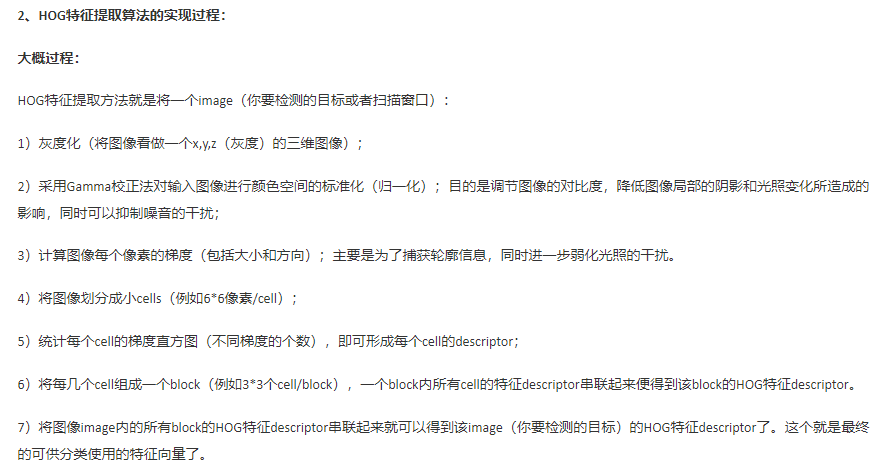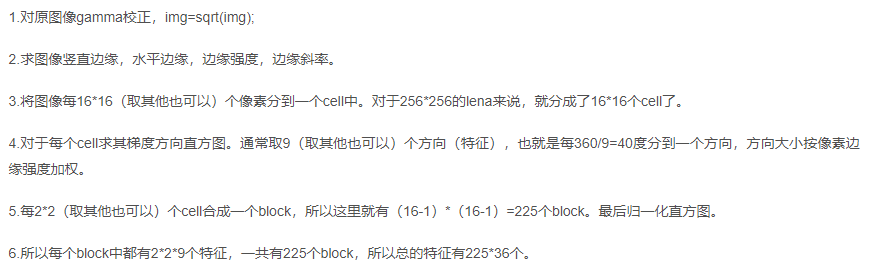7、朴素贝叶斯算法的推导过程（特征独立、公式写全）

8、决策树学习包括三个步骤（特征选择（经验熵与经验条件熵之差）、决策树生成和决策树修剪）

9、决策树是什么？

10、决策树与条件概率分布的关系？（决策树表示给定特征条件下类的条件概率分布，这一条件概率分布定义在特征空间的一个划分上，将特征空间划分成互不相交的单元或者区域，并在每个单元定义一个类的概率分布就构成了一个条件概率分布，决策树的一条路径对应于划分中的一个单元。决策树所表示的条件概率分布由各个单元给定条件下类的条件概率分布组成）

11、 决策树模型是条件概率模型，决策树损失函数是正则化的极大似然函数（损失函数后面加了a|T|项，用于调节模型复杂度与训练数据拟合之间的平衡关系），学习策略是以损失函数为目标函数的最小化，学习算法采用启发式方法近似求解最优化问题。

12、决策树的学习过程是怎么样（递归的选择最优特征）？

13、决策树特征选择的准则是什么？（信息增益g(D,A)=H(D)-H(D|A)与信息增益比gR=g(D,A)/HA(D),信息增益是得知特征X的信息而使得类Y的信息的不确定减少的程度）

14、 ID3算法（用信息增益进行特征选择），C4.5算法（用信息增益比进行特征选择）

15、CART分类回归树，回归树用平方误差最小化，分类树用基尼指数最小化准则（样本集合的不确定性越小），进行特征选择，生成二叉树，剪枝得到的子序列采用了交叉验证法选取最优子树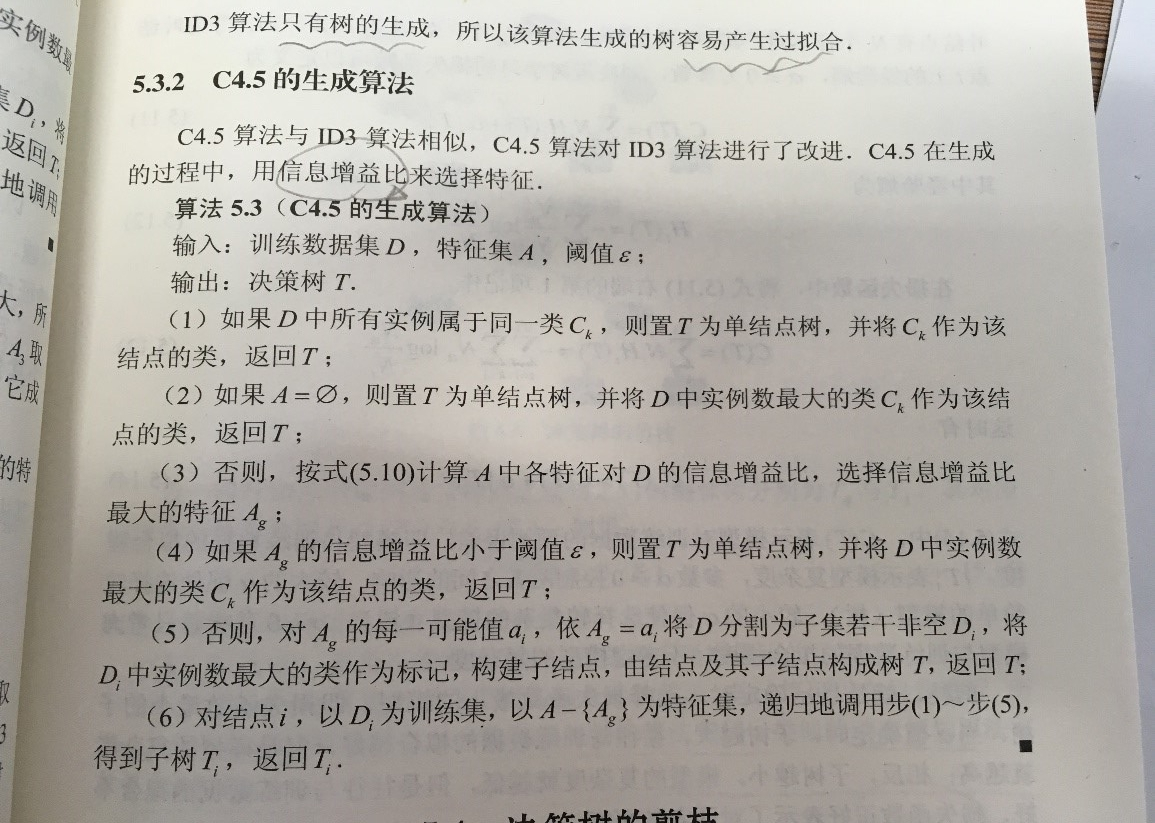16、逻辑斯蒂回归模型是条件概率分布，逻辑斯蒂模型中，输出Y=1的对数几率（事件发生的概率与不发生概率的比值）是由输入x的线性函数表示的模型。模型参数估计采用了极大似然估计法，逻辑斯蒂回归学习中通常采用的方法是梯度下降法和拟牛顿法。

17、过拟合有哪几种方法？分别是怎么处理的？

18、介绍下逻辑回归http://www.cnblogs.com/ModifyRong/p/7739955.html

19、什么是核技巧？

20、SVM
https://blog.csdn.net/xwchao2014/article/details/47948063
SVM中C越大则为了降低整体的目标函数，必须使得ζ越小，这必然会使得1-ζ越大，说明松弛条件更加严格，则说明分类更加严格。
https://blog.csdn.net/cppjava_/article/details/68060439
https://blog.csdn.net/xwchao2014/article/details/47948063
http://www.thpffcj.com/2018/05/22/Python-Machine-Learning-8/

21、SVM没有处理缺失值的策略（决策树有）
https://blog.csdn.net/u012328159/article/details/79413610
https://www.cnblogs.com/ljygoodgoodstudydaydayup/p/7418851.html

https://blog.csdn.net/xwchao2014/article/details/47953353

23、Kmeans算法流程以及优缺点
https://blog.csdn.net/u013719780/article/details/78413770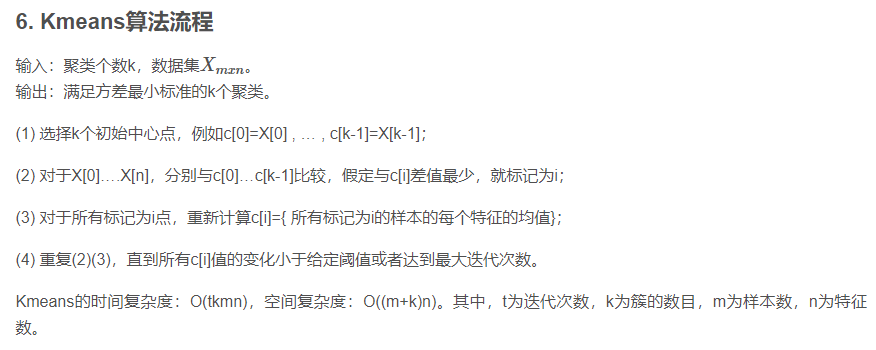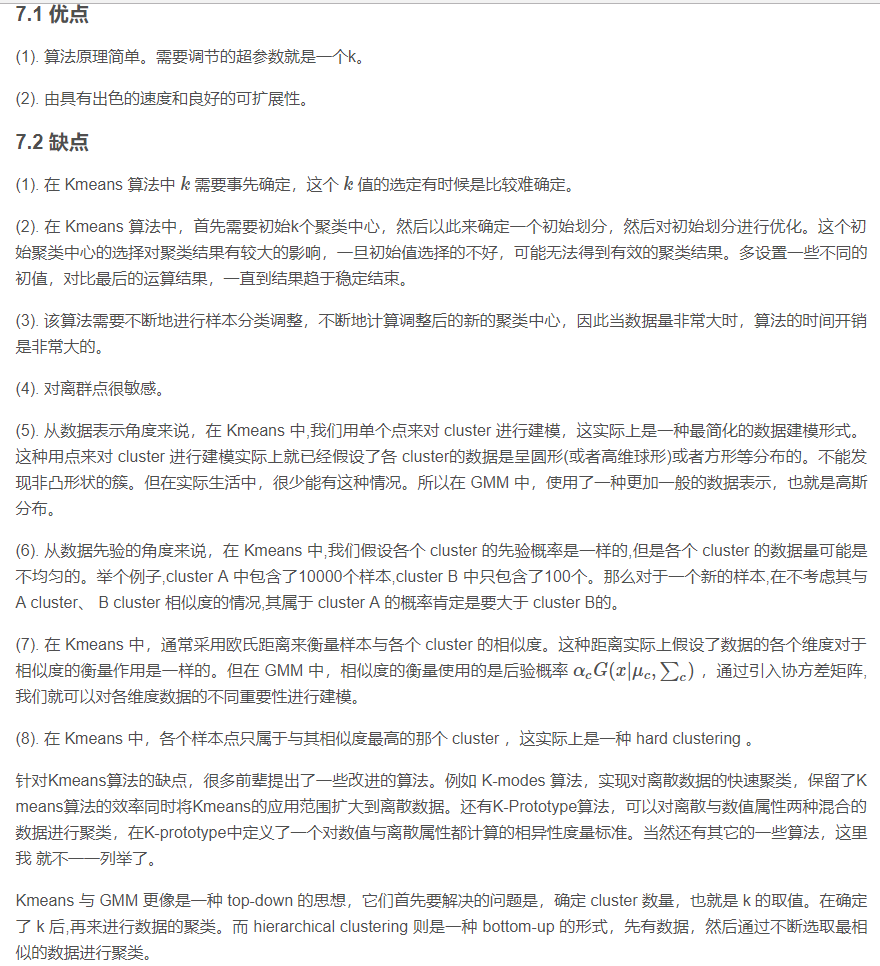k线 机器学习 相关内容

2018-12-15 15:21:05 qq_43604520 阅读数 55
• ###### 机器学习入门30天实战

购买课程后，可扫码进入学习群，获取唐宇迪老师答疑 系列课程包含Python机器学习库，机器学习经典算法原理推导，基于真实数据集案例实战3大模块。从入门开始进行机器学习原理推导，以通俗易懂为基础形象解读晦涩难懂的机器学习算法工作原理，案例实战中使用Python工具库从数据预处理开始一步步完成整个建模工作！具体内容涉及Python必备机器学习库、线性回归算法原理推导、Python实现逻辑回归与梯度下降、案例实战，信用卡欺诈检测、决策树与集成算法、支持向量机原理推导、SVM实例与贝叶斯算法、机器学习常规套路与Xgboost算法、神经网络。

7599 人正在学习 去看看 唐宇迪

## 机器学习

1.监督学习

2.无监督学习

3.过拟合：训练集好，测试集不好。(后期再详细介绍）

# 单变量线性回归

1.代价函数（先算损失函数，再用代价函数J优化参数值，损失函数越接近0越好，损失函数即方差【也可以再乘与0.5】）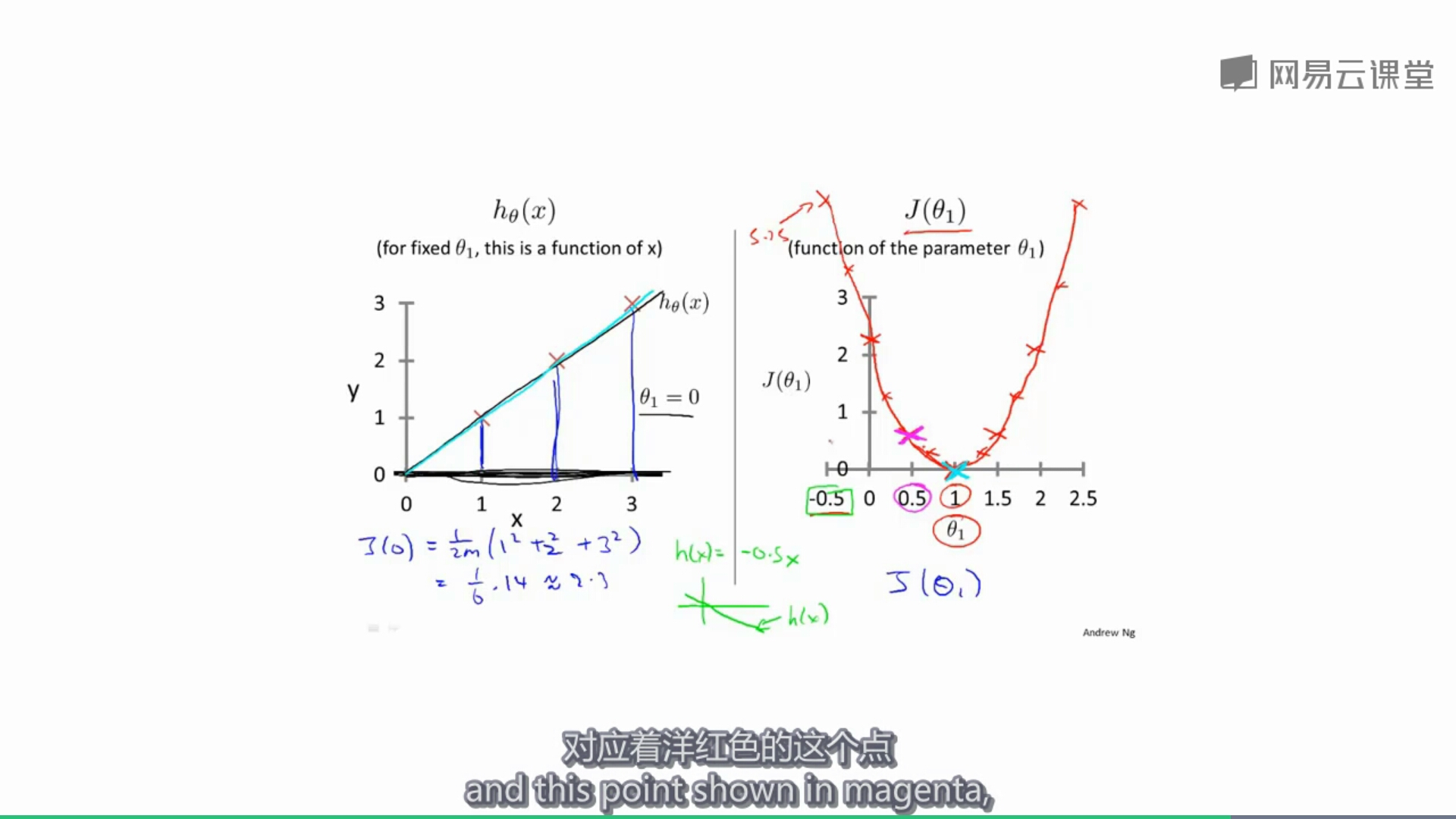1.通过降低J（k）；J(k，b）的值，找到最小的j值，或全局最小。（公式：下面图中有）
2.当k，b的起始值不同时，得到最后的最优值也可能不同。
3.k，b同时变换，如果已经在局部最优则不再变换。
4.随着k值的减小，移动幅度越来越慢，学习率不用改变。
5.学习率α不易过大或过小：过小接近最优处速度较慢，过大可能会移动幅度太大跃过最优值。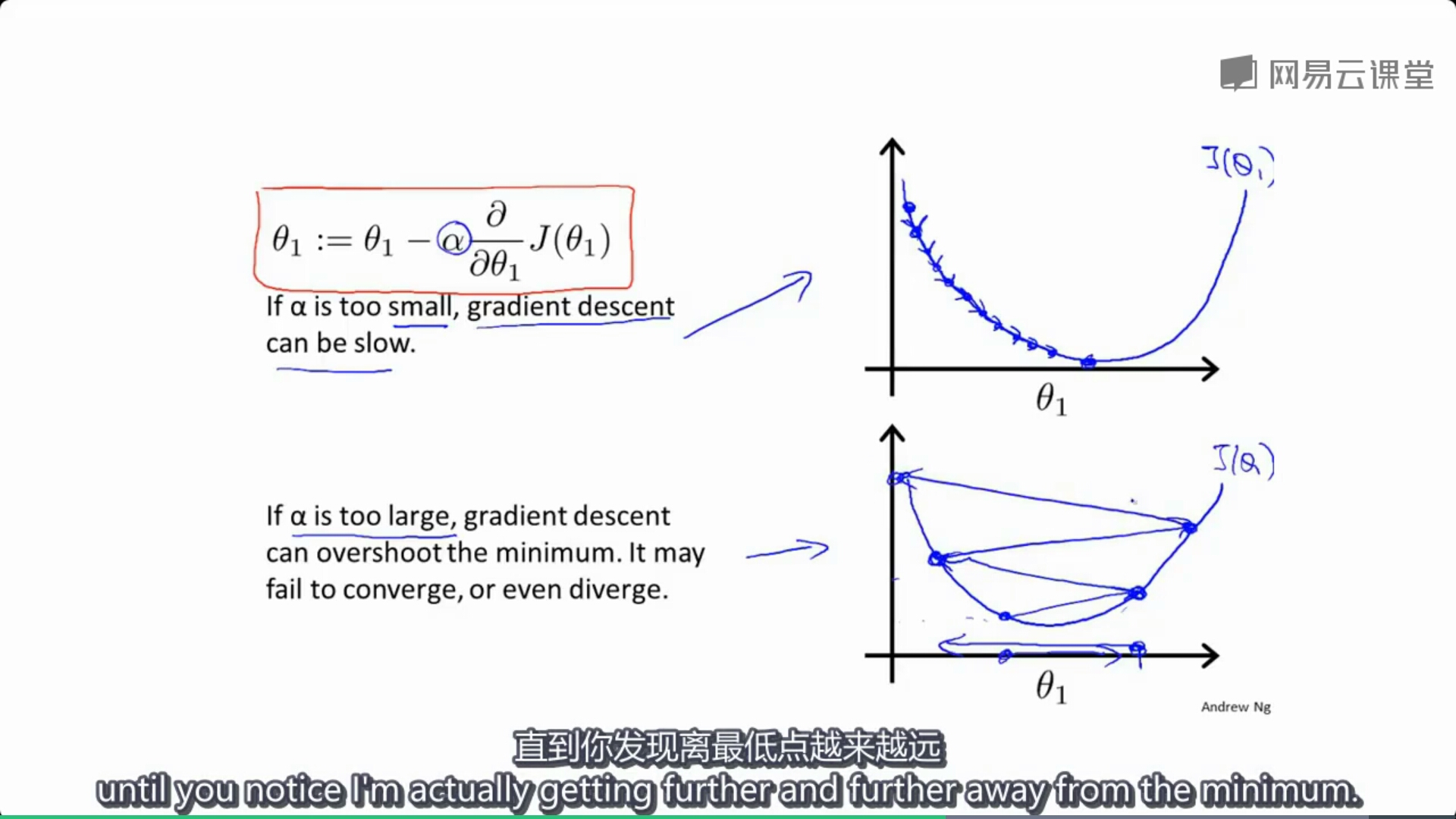6.随着越来越接近局部最优值，移动幅度会逐渐变小，α的值不用改变。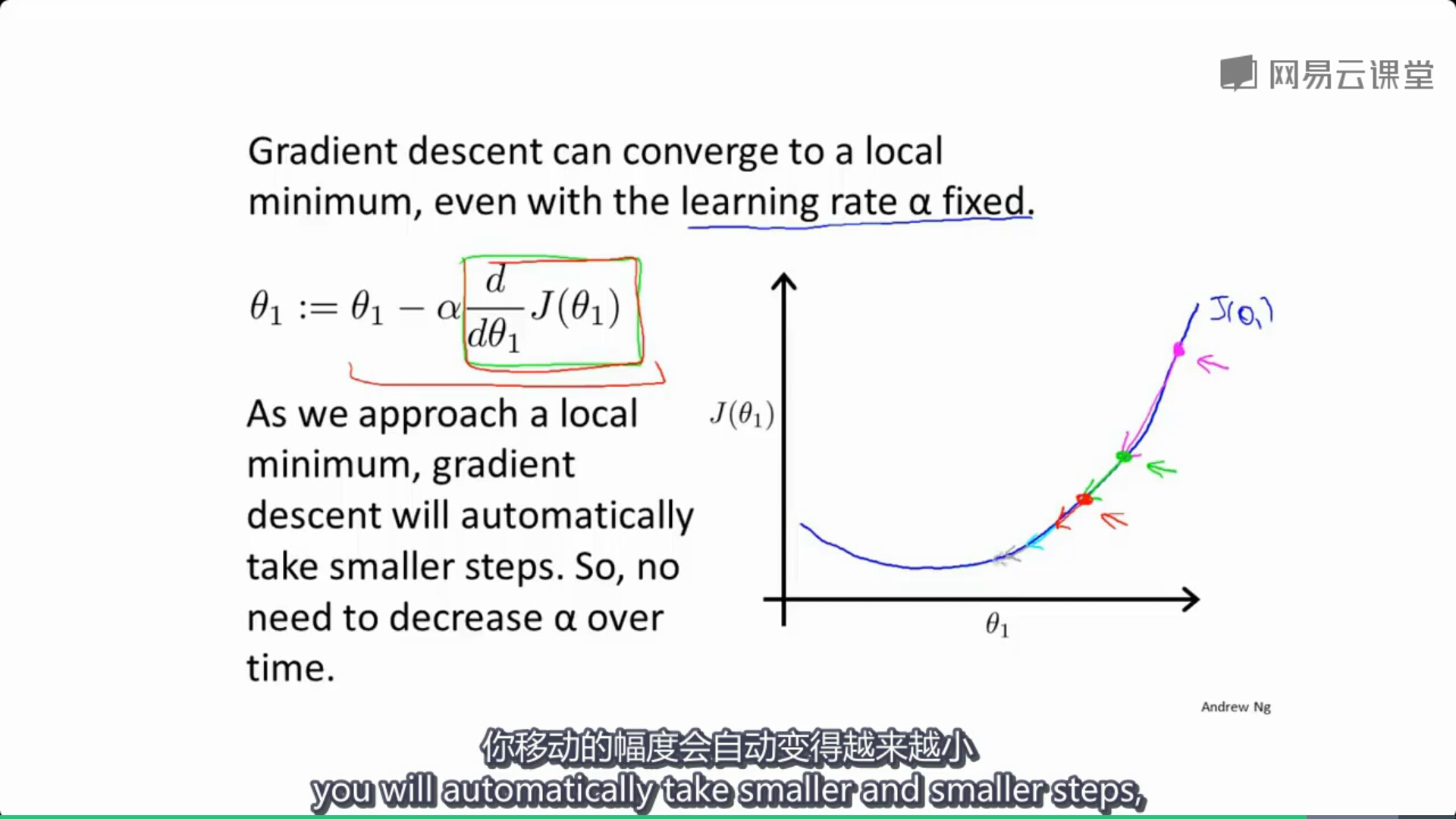# 矩阵

1.矩阵加法，减法：（个人理解）就是相同位置上的数相加减，行列数要相同。

2.乘法：口头不好描述，上图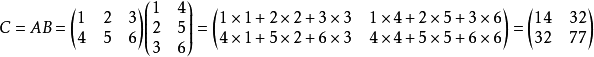3.矩阵不遵守乘法交换率（与单位矩阵相乘除外），遵守乘法结合律。

4.矩阵逆运算：两个矩阵相乘等于单位矩阵。则一个矩阵称为另一个矩阵的逆矩阵。

5.矩阵转置运算：横着的矩阵翻转变成竖着。翻转后：左上对左上，左下对右上。

## 多变量线性回归

1.有多个变量，例如估算房价，面积，房间数，房龄…

2.可以用矩阵来代替公式，较简单。取个x0=1.

3.代价函数J（θ）的下降。

4.特征缩放：由于当不同变量数值先插过大时会经过很长时间才能算出结果，因此可以让变量除以一个数，让变量在[-1,1]之间（这只是一个近似值），最好是不要超出[-3,3]和[-1\3,1\3].

5.多元梯度下降：

k线 机器学习 相关内容